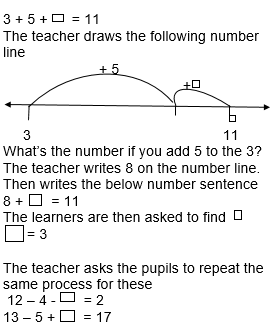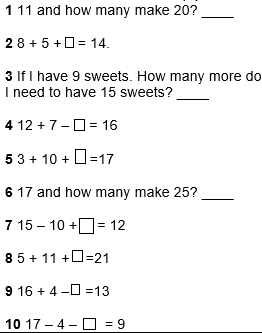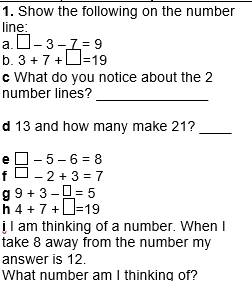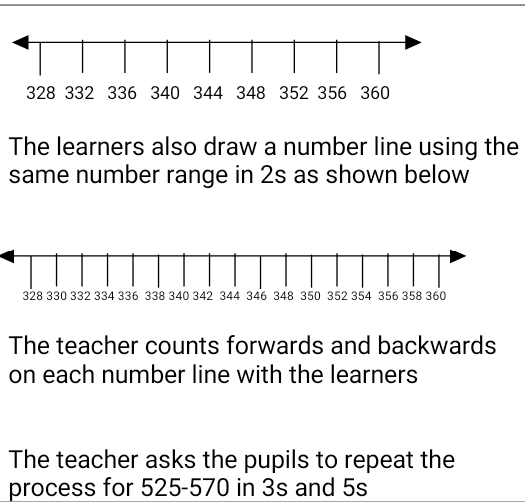# Lesson Notes By Weeks and Term - Primary 3

TERM: 1st Term

WEEK: 9

CLASS: Primary 3

AGE: 8 years

DURATION: 5 periods of 40 minutes each

DATE:

SUBJECT: Mathematics

SPECIFIC OBJECTIVES: At the end of the lesson, the pupils should be able to

1. Find the missing number using addition and subtraction as inverse operations.

INSTRUCTIONAL TECHNIQUES: Explanation, question and answer, demonstration, practical, assessments

INSTRUCTIONAL MATERIALS: Base 10 kit, 100 number board, scrap paper, videos from source-

PERIOD 1: What is the missing number?

 PRESENTATION TEACHER’S ACTIVITY PUPIL’S ACTIVITY STEP 1MENTAL MATHS The teacher begins the lesson with some mental calculationsCalculate1. 13 - 4 =2. 14 - 5 =3. 14 - 6 =4. 15 - 6 =5. 15 - 7 =6. 16 - 7 =7. 17 - 8 =8. 17 - 9 =9. 16 - 8 =10. 18 - 9 = Pupils respond and participate STEP 2CONCEPTDEVELOPMENT The teacher asks the learners the following question“ 4 and how many make 12?” The teacher discusses with the learners how they got the answer whether they used addition or subtraction The teacher writes the following problem on the board “There were 3 fish in a bucket. Sesan put 5 fish in it, then Bayo put some more fish. There are now 11 fish in the bucket. How many fish did Bayo put?”The teacher writes a number sentence representing the situation by explaining the word problemPupils pay attention and participate STEP 3CLASS-WORK Complete the following. You may want to draw a number line to help you.Pupils attempt their class work STEP 4HOME-WORKPupils attempt their class work STEP 5SUMMARY The teacher summarizes reminding the pupils that we can use subtraction to solve addition problems She marks their class works, makes corrections where necessary and commends them positively

PERIOD 2: What’s the missing number?

 PRESENTATION TEACHER’S ACTIVITY PUPIL’S ACTIVITY STEP 1ORALASSESSMENTS The teacher asks questions on mental maths with 2 and 3-digit numbers treated so far Pupils respond and participate STEP 2DISCUSSION The teacher discusses all the methods used by some learners in the oral assessments(some of the questions are solved on the board by the learners) and addresses any misconceptions that may have risen Pupils pay attention and participate STEP 3WRITTEN ASSESSMENTSPupils attempt their class work STEP 4SUMMARY The teacher marks the written assessments, corrects were necessary and commends the pupils

PERIOD 3: Counting in 2’s and 4’s

 PRESENTATION TEACHER’S ACTIVITY PUPIL’S ACTIVITY STEP 1MENTAL MATHS The teacher begins the lesson with some mental calculations Count forward in 2’s from 2 to 40, in 3’s from 3 to 30, in 4’s from 4 to 40, in 5’s from 5 up to 100 Pupils respond and participate STEP 2CONCEPTDEVELOPMENT The teacher asks the learners to draw the number line from 328 – 360 in 4’s as shown belowPupils pay attention and participate STEP 3CLASS-WORK 1 Extend the pattern:a 112, 116, 120, _____, _____, _____b 116, 112, 108, _____, _____, _____c 164, 162, 160, _____, _____, _____d 132, 129, 126, _____, _____, _____ 2 Draw the following number lines:a 4s from 492–528b 3s from 492–528c Which numbers are in both the 3s pattern and the 4s pattern? Pupils attempt their class work STEP 4HOME-WORK Complete the pattern:1 100, ____, 108, ____, 116, ____2 100, _____, 104, _____, 108, _____, 1123 Which numbers are in both the 2s pattern and the 4s pattern? The pupils writes it in their homework book STEP 5SUMMARY The teacher summarizes by reminding the pupils how to count in 2’s and 4’s and more importantly the number patterns and relationship between 2’s and 4’s She marks the class work, make corrections where necessary and commends the pupils positively

PERIOD 4: Counting in 20s, 25s, 50s and 100s

 PRESENTATION TEACHER’S ACTIVITY PUPIL’S ACTIVITY STEP 1MENTAL MATHS The teacher begins the lesson with some mental calculationsCount forwards from 20 in 20s, from 25 in 25s, from 50 in 50s up to 200 Pupils respond and participate STEP 2CONCEPTDEVELOPMENT The teacher asks the learners to draw number lines from 600 to 900 in 20s and 25sIn 20s,The teacher counts forwards and backwards on each number line with the learnersThe teacher then asks which number line can be used in counting in 50s(25s number line) She also asks which number line can be used to count in 100s(20s number line) The teacher asks the pupils what is common between the 20s and 25s number line(600, 700, 800, 900) CLASS ACTIVITYThe learners are asked to use the number line to answer the following questionsa. What’s common if I count in 20s and 25s from 300-500?b. what’s common if I count in 20s and 50s from 300 to 500?c. what’s common if I count in 50s and 100s from 600 to 800?d. What common if I count in 20s, 25s, 50s and 100s from 100-900? Pupils pay attention and participate STEP 3CLASS-WORK 1 Describe these patterns:a 200, 220, 240, 260, 280, 300.320, 340, 360, 380, 400b 200, 225, 250, 275, 300, 325, 350, 375, 400c 200, 250, 300, 350, 400d 200, 300, 400e What’s common if I count in 20s and 25s from 200 to 400?f What’s common if I count in 25s and 50s from 200 to 400?g What’s common if I count in 50s and 100s from 200 to 400?h What’s common if I count in 20s and 100s from 200 to 400?i What’s common if I count in 20s, 25s, 50s and 100s from 200 to 400? 2 Extend these patterns:a 499, 494, 489, ___, ___, ___b 380, 360, 340, ___, ___, ___c 500, 400, 300, ___, ___, ___d 236, 336, 436, ___, ___, ___ Pupils attempt their class work STEP 4HOME-WORK Extend these patterns:1 300, 400, ____2 300, 350, ___, 450, ___3 300, 325, 350, ___, 400, ___, 450, ___, 5004 What is common between 1) and 2)?5 What is common between 2) and 3)?6 What is common between 1) and 3)? The pupils writes it in their homework book STEP 5SUMMARY The teacher summarizes by reminding the pupils how to count in 20s, 25s, 50s and 100s and the number patterns and relationships between them She marks the class work, make corrections where necessary and commends the pupils positively

PERIOD 5: Weekly Test/consolidations

TEACHER’S ACTIVITY: The teacher revises all the concepts treated from period 1-4 and gives the pupils follow through exercises, quiz and tests . She marks the exercises, makes corrections and commends the pupils positively.

PUPIL’S ACTIVITY: The pupils work on the worksheets and exercises given by the teacher individually

ASSESSMENT

1 Describe these patterns:

a 100, 102, 104, 106, 108

b 360, 370, 380, 390, 400,

c 414, 417, 420, 423

2 Extend these patterns:

a 199, 299, 399, ____, ____

b 580, 560, 540, ___, ___, ___

c 450, 500, 550, ___, ___, ___

3 Draw the following number lines:

a 5s from 720–765

b 3s from 720–765

c Which numbers are in both the 5s pattern and the 3s pattern?

4 Complete the patterns:

a 127, 130, 133, ___, ___, ___

b 108, 105, 102, __, __, __

c ___, ___, ___, 198, 195, 192

d 38, 40, 42, ___, ___, ___

e 140, ___, 144, ___, 148, _____